Скачать презентацию 1 CHAPTER IV GROWTH AND ACCUMULATION 2

ch_4_ppp.ppt

• Размер: 273 Кб
• Количество слайдов: 30

## Описание презентации 1 CHAPTER IV GROWTH AND ACCUMULATION 2 по слайдам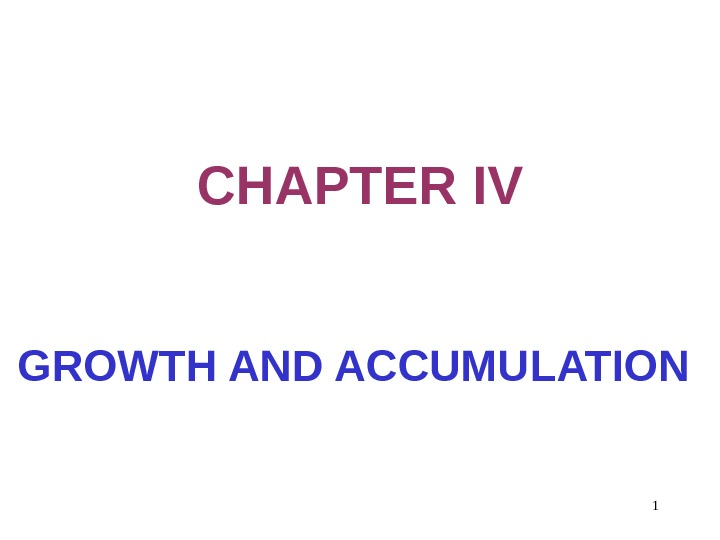1 CHAPTER IV GROWTH AND ACCUMULATION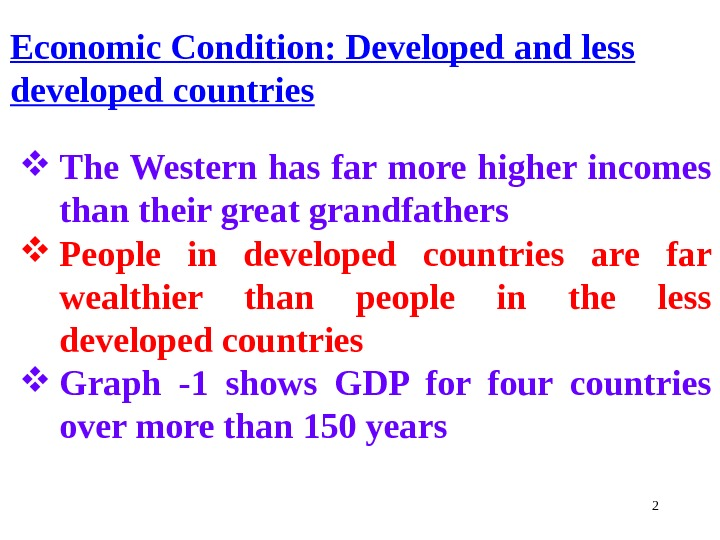2 Economic Condition: Developed and less developed countries The Western has far more higher incomes than their great grandfathers People in developed countries are far wealthier than people in the less developed countries Graph -1 shows GDP for four countries over more than 150 years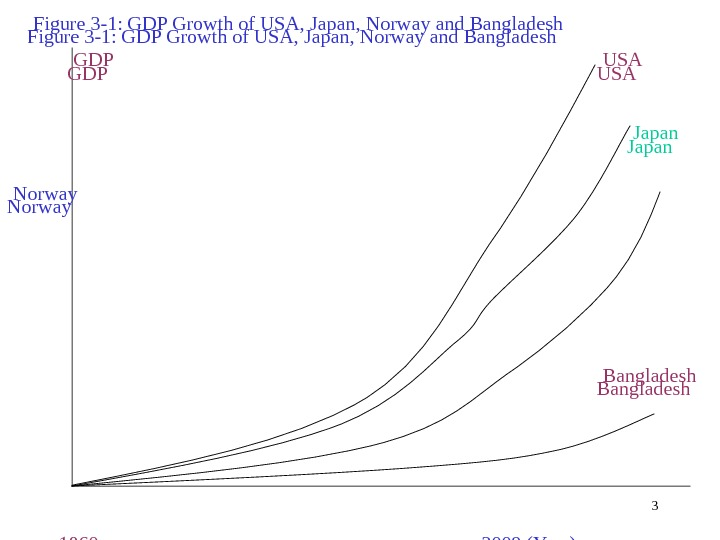3 Figure 3 -1: GDP Growth of USA, Japan, Norway and Bangladesh GDP USA Japan Norway Bangladesh 1860 2009 (Year)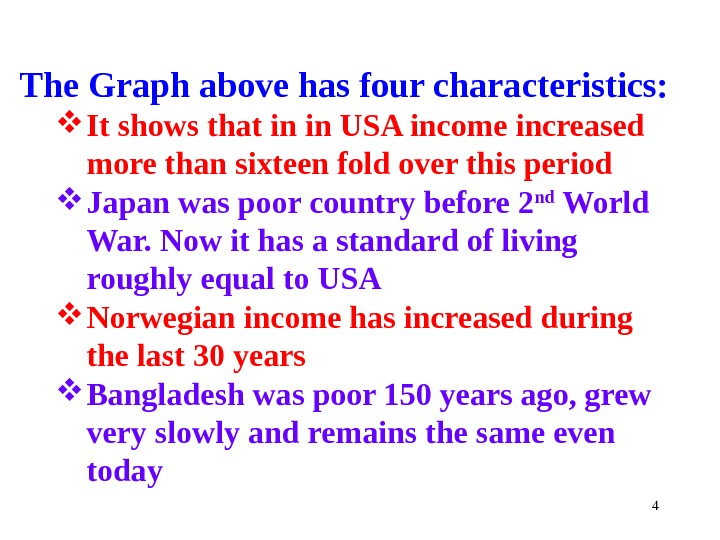4 The Graph above has four characteristics: It shows that in in USA income increased more than sixteen fold over this period Japan was poor country before 2 nd World War. Now it has a standard of living roughly equal to USA Norwegian income has increased during the last 30 years Bangladesh was poor 150 years ago, grew very slowly and remains the same even today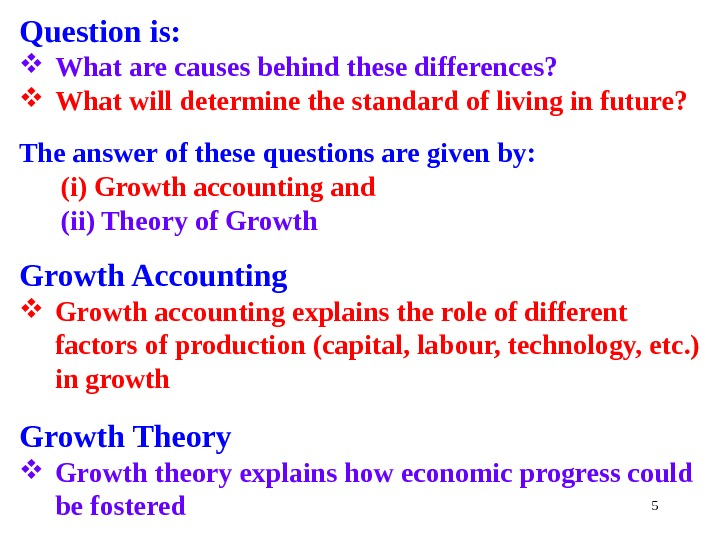5 Question is: What are causes behind these differences? What will determine the standard of living in future? The answer of these questions are given by: (i) Growth accounting and (ii) Theory of Growth Accounting Growth accounting explains the role of different factors of production (capital, labour, technology, etc. ) in growth Growth Theory Growth theory explains how economic progress could be fostered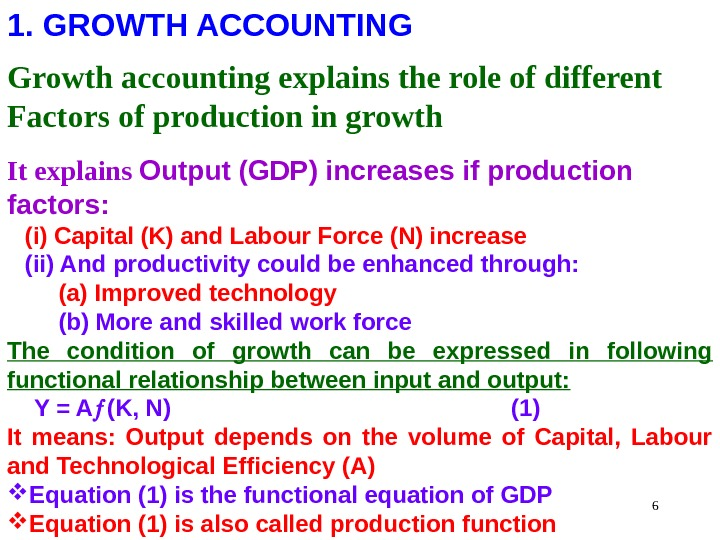61. GROWTH ACCOUNTING Growth accounting explains the role of different Factors of production in growth It explains Output (GDP) increases if production factors: (i) Capital (K) and Labour Force (N) increase (ii) And productivity could be enhanced through: (a) Improved technology (b) More and skilled work force The condition of growth can be expressed in following functional relationship between input and output: Y = A (K, N) (1) It means: Output depends on the volume of Capital, Labour and Technological Efficiency (A) Equation (1) is the functional equation of GDP Equation (1) is also called production function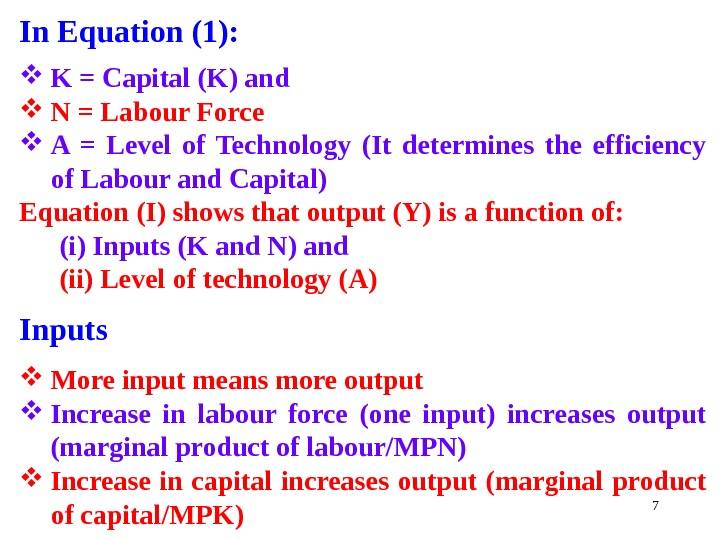7 In Equation (1): K = Capital (K) and N = Labour Force A = Level of Technology (It determines the efficiency of Labour and Capital) Equation (I) shows that output (Y) is a function of: (i) Inputs (K and N) and (ii) Level of technology (A) Inputs More input means more output Increase in labour force (one input) increases output (marginal product of labour/MPN) Increase in capital increases output (marginal product of capital/MPK)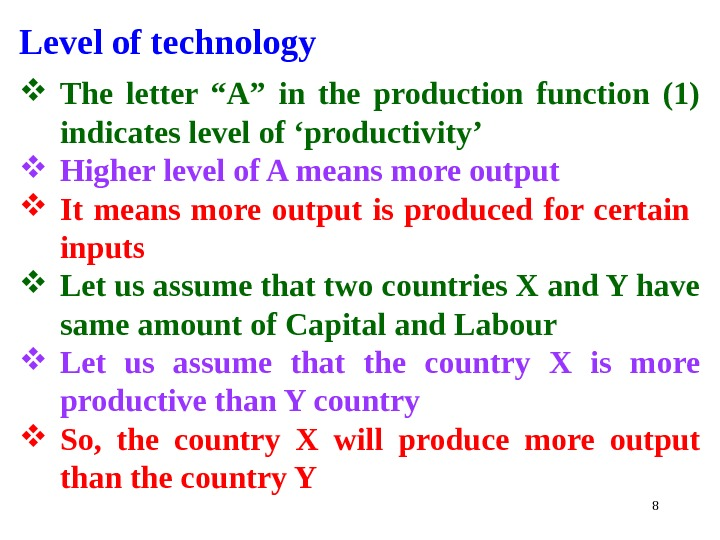8 Level of technology The letter “A” in the production function (1) indicates level of ‘productivity’ Higher level of A means more output It means more output is produced for certain inputs Let us assume that two countries X and Y have same amount of Capital and Labour Let us assume that the country X is more productive than Y country So, the country X will produce more output than the country Y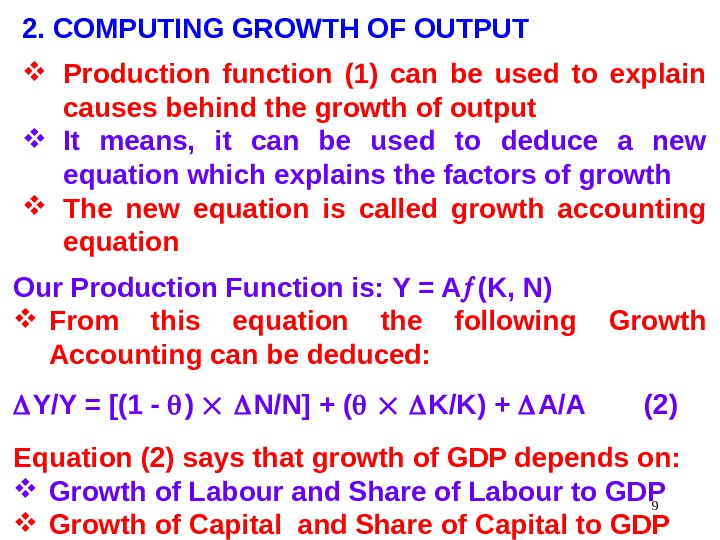92. COMPUTING GROWTH OF OUTPUT Production function (1) can be used to explain causes behind the growth of output It means, it can be used to deduce a new equation which explains the factors of growth The new equation is called growth accounting equation Our Production Function is: Y = A (K, N) From this equation the following Growth Accounting can be deduced: Y/Y = [(1 — ) N/N] + ( K/K) + A/A (2) Equation (2) says that growth of GDP depends on: Growth of Labour and Share of Labour to GDP Growth of Capital and Share of Capital to GDP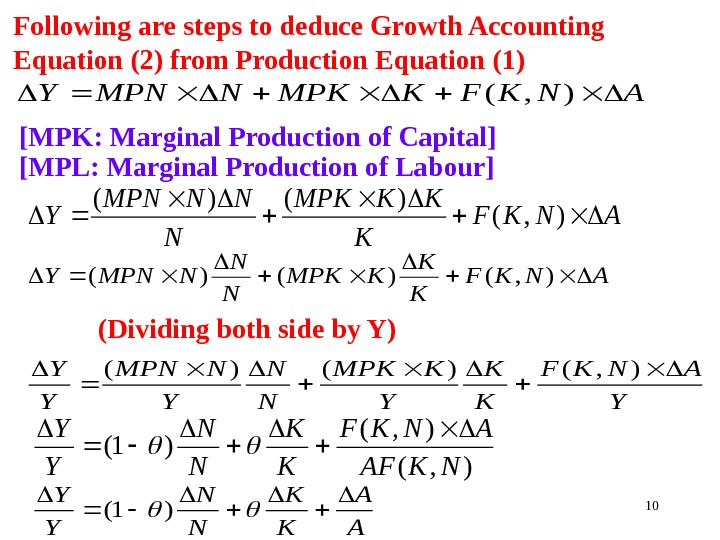10 (Dividing both side by Y)ANKFKMPKNMPNY), ( ANKF K K KMPK N N NMPNY ), ()()( Y ANKF K K Y KMPK N N Y NMPN Y Y ), ()()( ), ( )1( NKAF ANKF K K N N Y Y A A K K N N Y Y )1( ANKF K KKMPK N NNMPN Y ), ( )()(Following are steps to deduce Growth Accounting Equation (2) from Production Equation (1) [MPK: Marginal Production of Capital] [MPL: Marginal Production of Labour]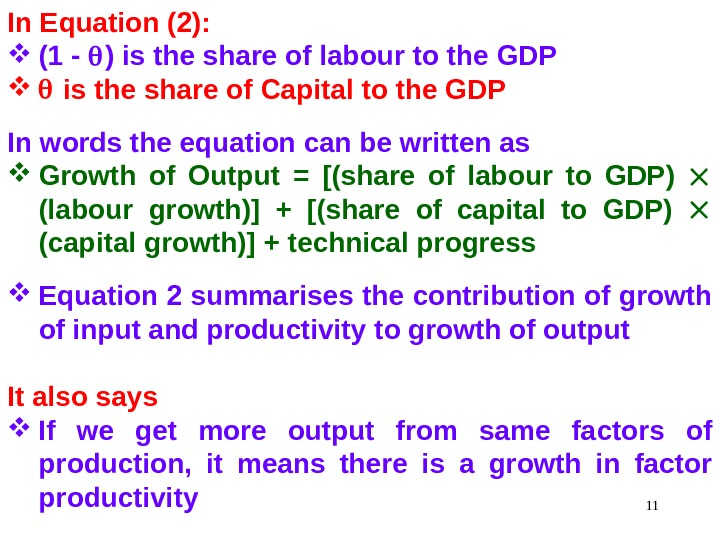11 In Equation (2): (1 — ) is the share of labour to the GDP is the share of Capital to the GDP In words the equation can be written as Growth of Output = [(share of labour to GDP) (labour growth)] + [(share of capital to GDP) (capital growth)] + technical progress Equation 2 summarises the contribution of growth of input and productivity to growth of output It also says If we get more output from same factors of production, it means there is a growth in factor productivity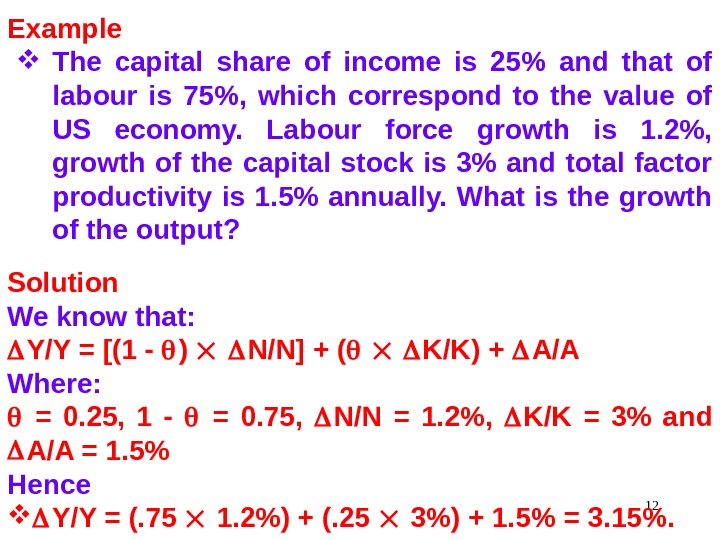12 Example The capital share of income is 25% and that of labour is 75%, which correspond to the value of US economy. Labour force growth is 1. 2%, growth of the capital stock is 3% and total factor productivity is 1. 5% annually. What is the growth of the output? Solution We know that: Y/Y = [(1 — ) N/N] + ( K/K) + A/A Where: = 0. 25, 1 — = 0. 75, N/N = 1. 2%, K/K = 3% and A/A = 1. 5% Hence Y/Y = (. 75 1. 2%) + (. 25 3%) + 1. 5% = 3. 15%.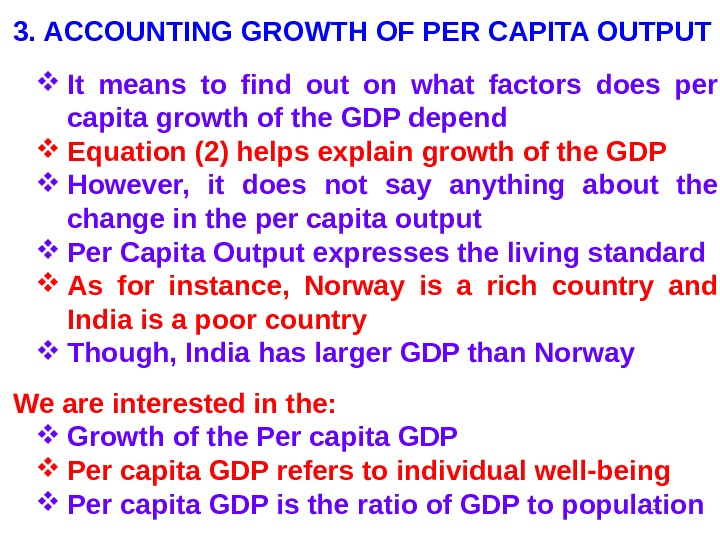133. ACCOUNTING GROWTH OF PER CAPITA OUTPUT It means to find out on what factors does per capita growth of the GDP depend Equation (2) helps explain growth of the GDP However, it does not say anything about the change in the per capita output Per Capita Output expresses the living standard As for instance, Norway is a rich country and India is a poor country Though, India has larger GDP than Norway We are interested in the: Growth of the Per capita GDP refers to individual well-being Per capita GDP is the ratio of GDP to population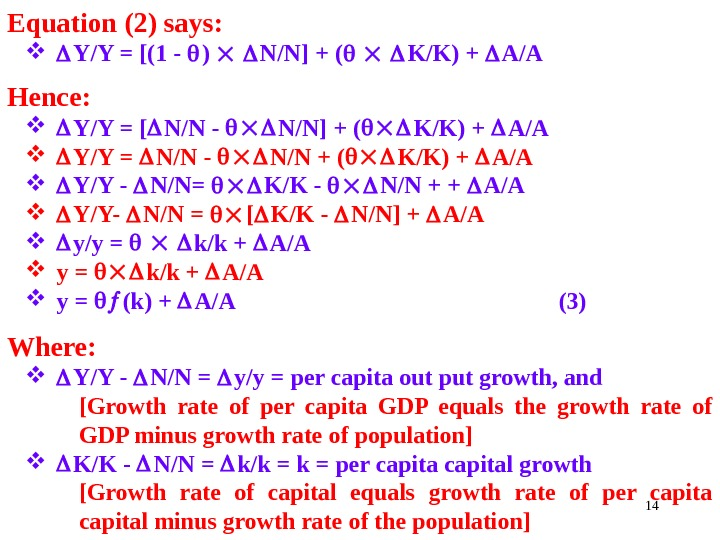14 Equation (2) says: Y/Y = [(1 — ) N/N] + ( K/K) + A/A Hence: Y/Y = [ N/N — N/N] + ( K/K) + A/A Y/Y = N/N — N/N + ( K/K) + A/A Y/Y — N/N= K/K — N/N + + A/A Y/Y- N/N = [ K/K — N/N] + A/A y/y = k/k + A/A y = k/k + A/A y = (k) + A/A (3) Where: Y/Y — N/N = y/y = per capita out put growth, and [Growth rate of per capita GDP equals the growth rate of GDP minus growth rate of population] K/K — N/N = k/k = per capital growth [Growth rate of capital equals growth rate of per capital minus growth rate of the population]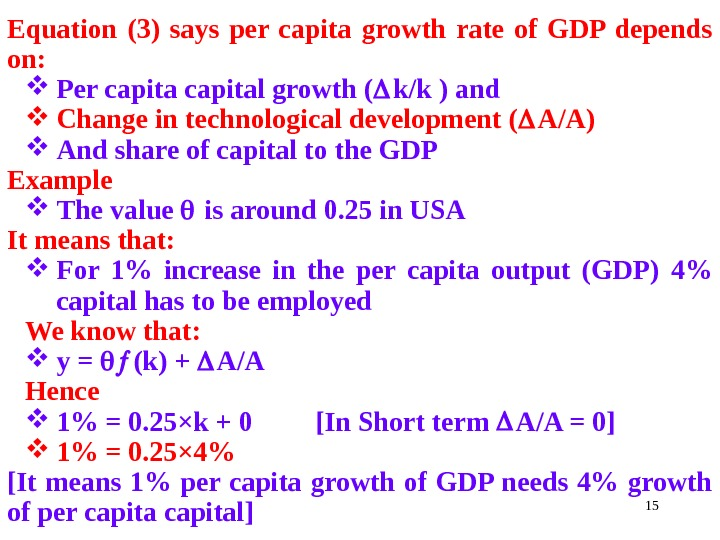15 Equation (3) says per capita growth rate of GDP depends on: Per capital growth ( k/k ) and Change in technological development ( A/A) And share of capital to the GDP Example The value is around 0. 25 in USA It means that: For 1% increase in the per capita output (GDP) 4% capital has to be employed We know that: y = (k) + A/A Hence 1% = 0. 25 ×k + 0 [In Short term A/A = 0] 1% = 0. 25 × 4% [It means 1% per capita growth of GDP needs 4% growth of per capital]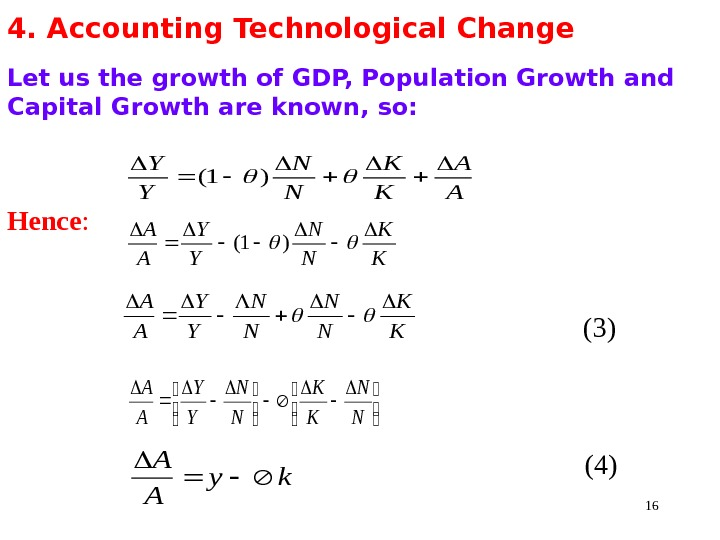164. Accounting Technological Change Let us the growth of GDP, Population Growth and Capital Growth are known, so: Hence : (3) (4)A A K K N N Y Y )1( K K N N Y Y A A N N K K N N Y Y A A ky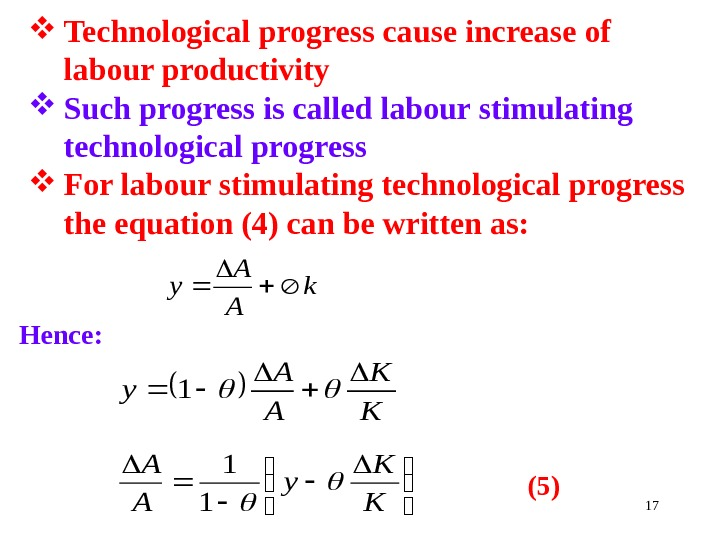17 Technological progress cause increase of labour productivity Such progress is called labour stimulating technological progress For labour stimulating technological progress the equation (4) can be written as: Hence: (5) K K A A y 1 K K y A A 1 1 k A A y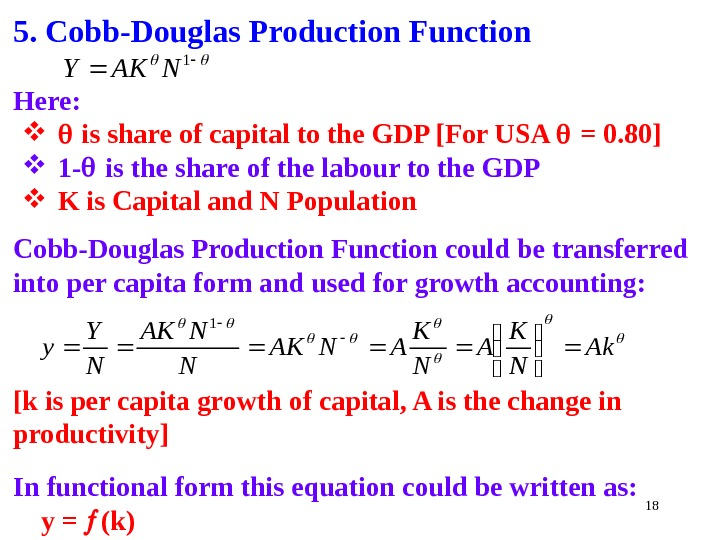185. Cobb-Douglas Production Function Here: is share of capital to the GDP [For USA = 0. 80] 1 — is t he share of the labour to the GDP K is Capital and N Population Cobb-Douglas Production Function could be transferred into per capita form and used for growth accounting: [k is per capita growth of capital, A is the change in productivity] In functional form this equation could be written as: y = (k) 1 NAKY Ak N K ANAK N Y y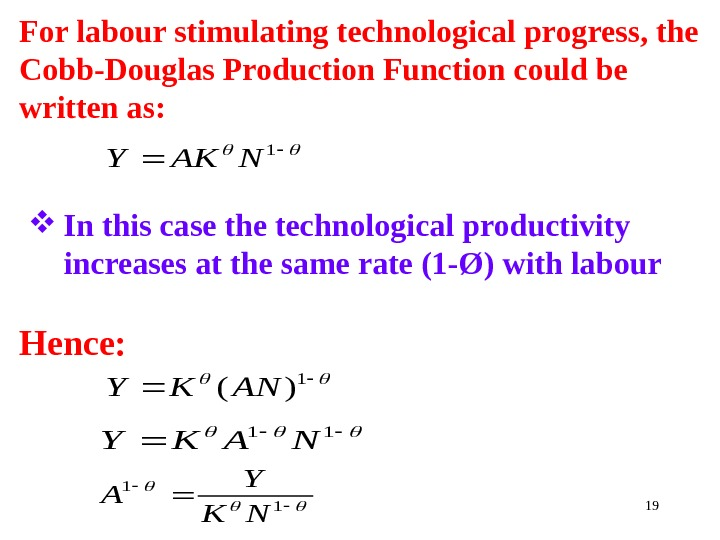19 For labour stimulating technological progress, the Cobb-Douglas Production Function could be written as: In this case the technological productivity increases at the same rate (1 — Ø) with labour Hence: 1 )(ANKY 1 NAKY 11 NAKY 1 1 NK Y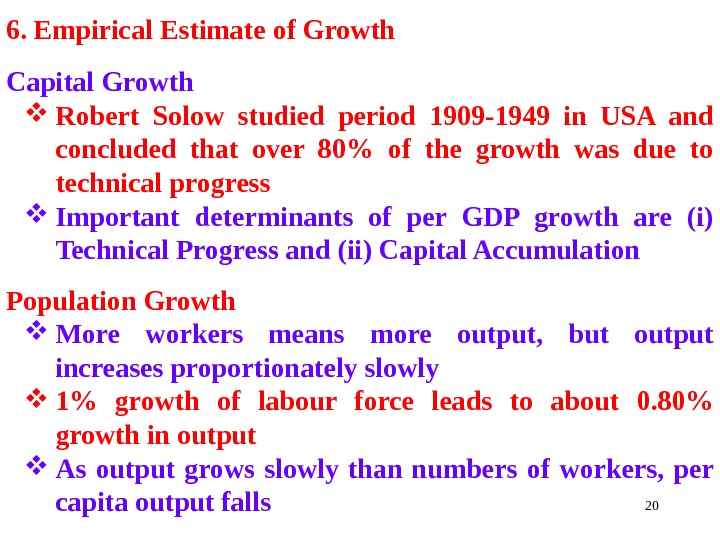206. Empirical Estimate of Growth Capital Growth Robert Solow studied period 1909 -1949 in USA and concluded that over 80% of the growth was due to technical progress Important determinants of per GDP growth are (i) Technical Progress and (ii) Capital Accumulation Population Growth More workers means more output, but output increases proportionately slowly 1% growth of labour force leads to about 0. 80% growth in output As output grows slowly than numbers of workers, per capita output falls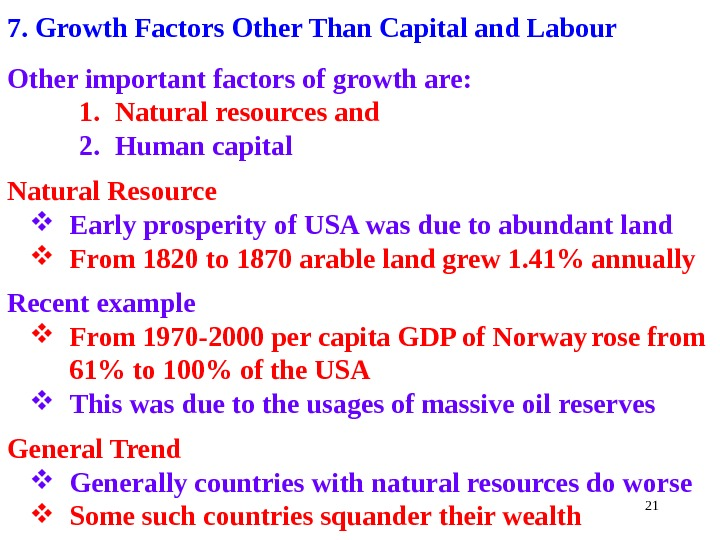217. Growth Factors Other Than Capital and Labour Other important factors of growth are: 1. Natural resources and 2. Human capital Natural Resource Early prosperity of USA was due to abundant land From 1820 to 1870 arable land grew 1. 41% annually Recent example From 1970 -2000 per capita GDP of Norway rose from 61% to 100% of the USA This was due to the usages of massive oil reserves General Trend Generally countries with natural resources do worse Some such countries squander their wealth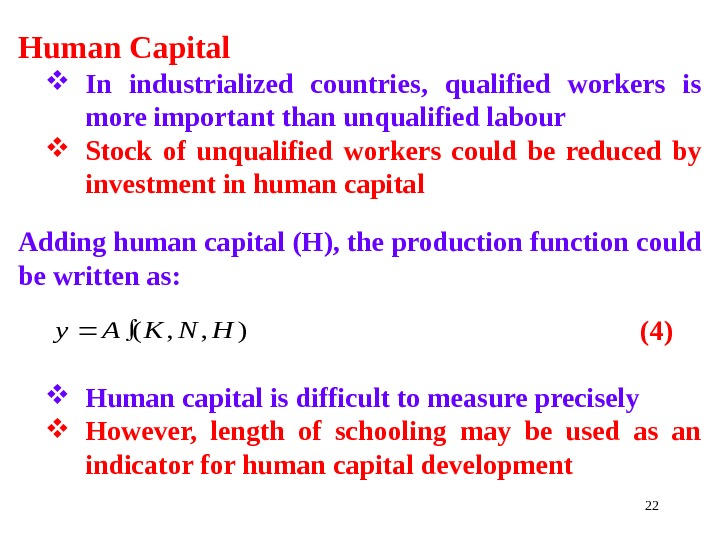22 Human Capital In industrialized countries, qualified workers is more important than unqualified labour Stock of unqualified workers could be reduced by investment in human capital Adding human capital (H), the production function could be written as: (4) Human capital is difficult to measure precisely However, length of schooling may be used as an indicator for human capital development), , (HNKAy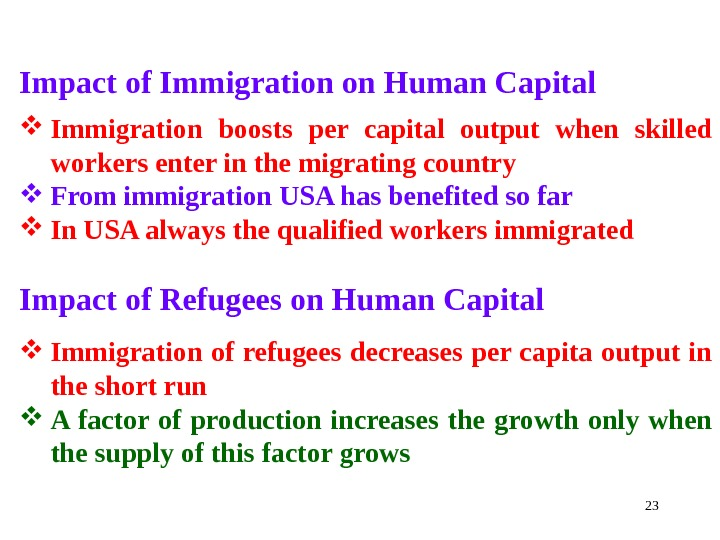23 Impact of Immigration on Human Capital Immigration boosts per capital output when skilled workers enter in the migrating country From immigration USA has benefited so far In USA always the qualified workers immigrated Impact of Refugees on Human Capital Immigration of refugees decreases per capita output in the short run A factor of production increases the growth only when the supply of this factor grows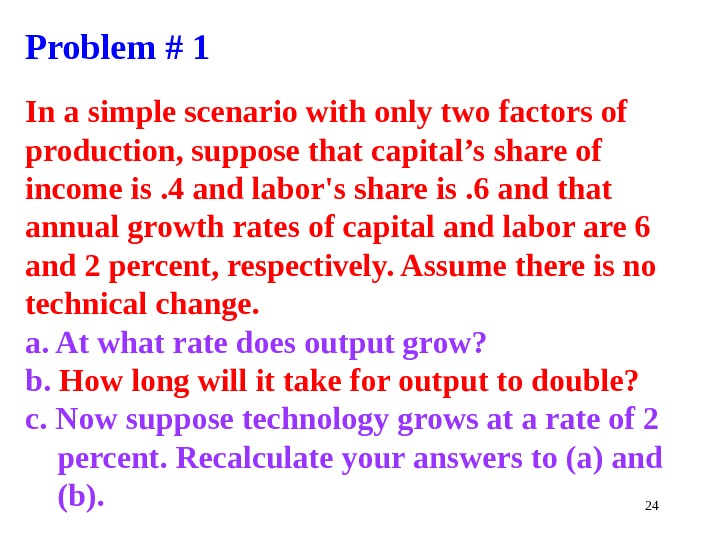24 Problem # 1 In a simple scenario with only two factors of production, suppose that capital’s share of income is. 4 and labor’s share is. 6 and that annual growth rates of capital and labor are 6 and 2 percent, respectively. Assume there is no technical change. a. At what rate does output grow? b. How long will it take for output to double? c. Now suppose technology grows at a rate of 2 percent. Recalculate your answers to (a) and (b).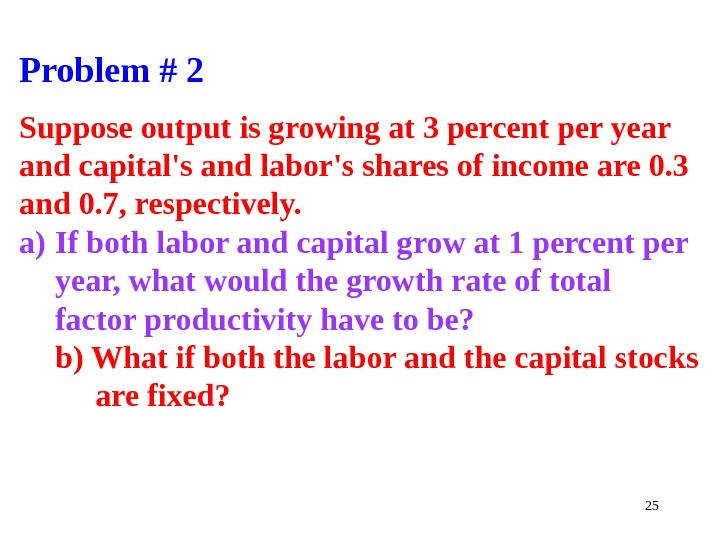25 Problem # 2 Suppose output is growing at 3 percent per year and capital’s and labor’s shares of income are 0. 3 and 0. 7, respectively. a) If both labor and capital grow at 1 percent per year, what would the growth rate of total factor productivity have to be? b) What if both the labor and the capital stocks are fixed?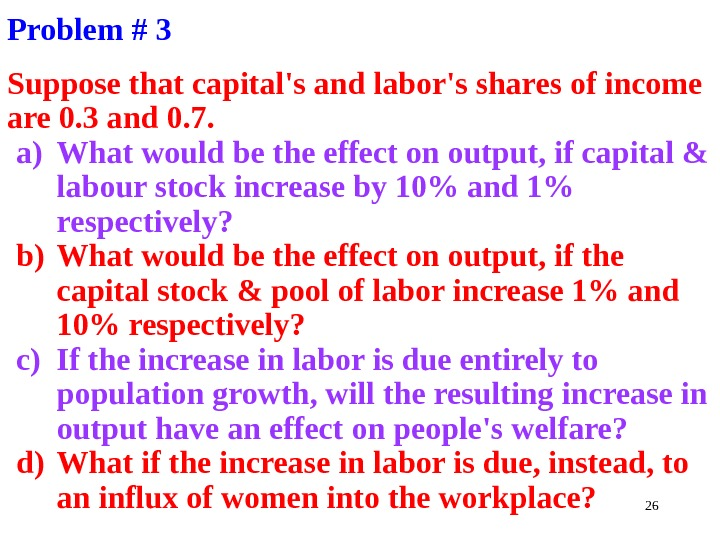26 Problem # 3 Suppose that capital’s and labor’s shares of income are 0. 3 and 0. 7. a) What would be the effect on output, if capital & labour stock increase by 10% and 1% respectively? b) What would be the effect on output, if the capital stock & pool of labor increase 1% and 10% respectively? c) If the increase in labor is due entirely to population growth, will the resulting increase in output have an effect on people’s welfare? d) What if the increase in labor is due, instead, to an influx of women into the workplace?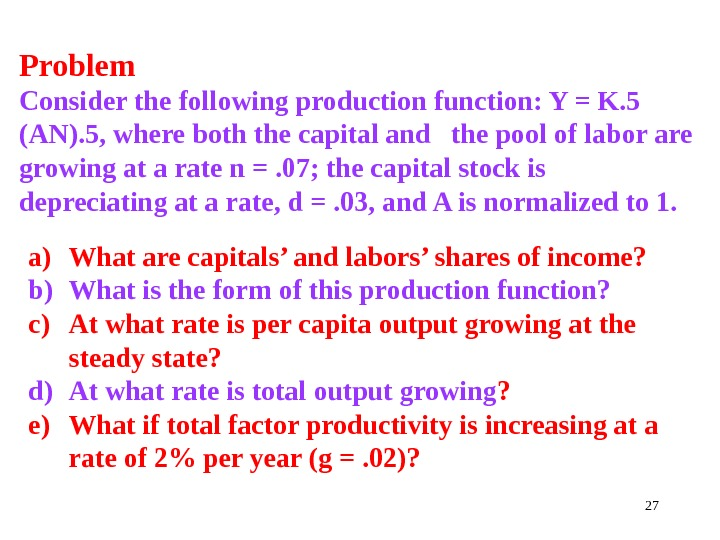27 Problem Consider the following production function: Y = K. 5 (AN). 5, where both the capital and the pool of labor are growing at a rate n =. 07; the capital stock is depreciating at a rate, d =. 03, and A is normalized to 1. a) What are capitals’ and labors’ shares of income? b) What is the form of this production function? c) At what rate is per capita output growing at the steady state? d) At what rate is total output growing ? e) What if total factor productivity is increasing at a rate of 2% per year (g =. 02)?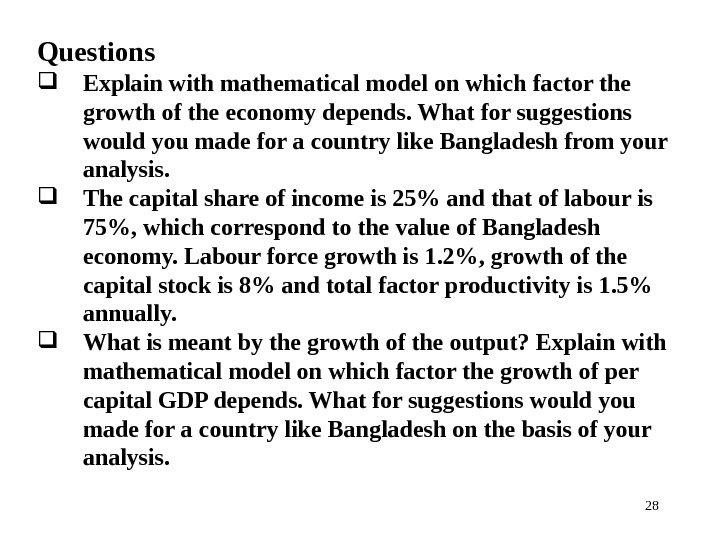28 Questions Explain with mathematical model on which factor the growth of the economy depends. What for suggestions would you made for a country like Bangladesh from your analysis. The capital share of income is 25% and that of labour is 75%, which correspond to the value of Bangladesh economy. Labour force growth is 1. 2%, growth of the capital stock is 8% and total factor productivity is 1. 5% annually. What is meant by the growth of the output? Explain with mathematical model on which factor the growth of per capital GDP depends. What for suggestions would you made for a country like Bangladesh on the basis of your analysis.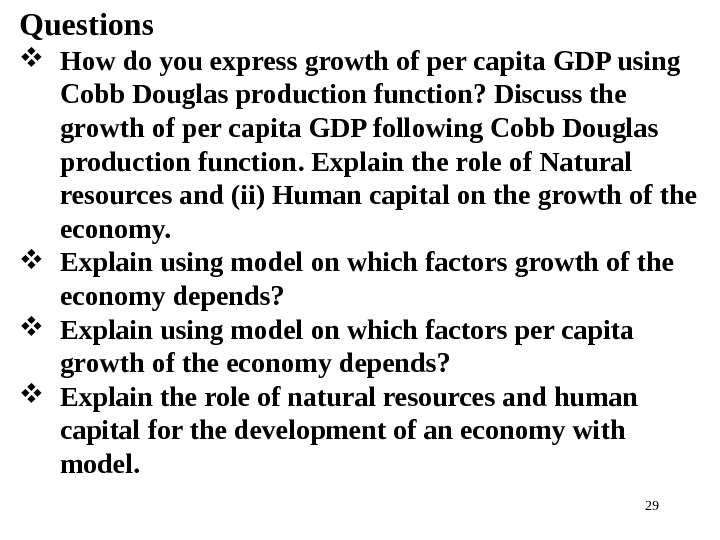29 Questions How do you express growth of per capita GDP using Cobb Douglas production function? Discuss the growth of per capita GDP following Cobb Douglas production function. Explain the role of Natural resources and (ii) Human capital on the growth of the economy. Explain using model on which factors growth of the economy depends? Explain using model on which factors per capita growth of the economy depends? Explain the role of natural resources and human capital for the development of an economy with model.30 End of the Chapter Tank You Very Much For Patient Listening